# Equations in Excel

Excel 365
Although Excel is used for calculating and presenting data, sometimes it is necessary to show the underlying formulas. Like Microsoft Word, Excel lets you use the Equation Editor to insert a beautifully designed math equation as a graphical object: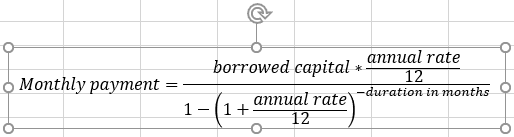Note: These equations do not perform actual calculations; they’re for display purposes only.

## Insert an equation in the Excel spreadsheet

To insert the equation in a spreadsheet, on the Insert tab, in the Symbols group, click the Equation button, then choose one of the equations from the gallery: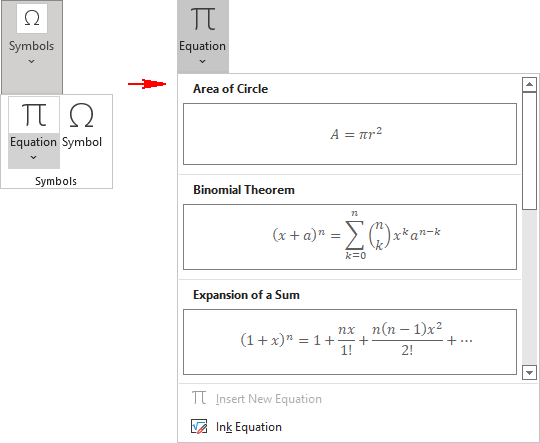The available predefined equations include:

• Area of Circle,
• Binomial Theorem,
• Expansion of a Sum,
• Fourier Series,
• Pythagorean Theorem,
• Taylor Expansion,
• Trig Identity 1,
• Trig Identity 2.

The equation is inserted into your worksheet: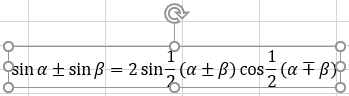## Create an equation

To create a new equation in a spreadsheet, on the Insert tab, in the Symbols group, click the Equation button: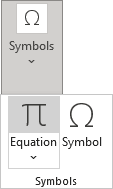Excel adds a new object in the middle of the spreadsheet (exactly in the center, not in the active cell) where you can insert the required symbols and letters: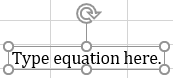## Working with equations

When you select an Equation object, you have access to the Shape Format tab: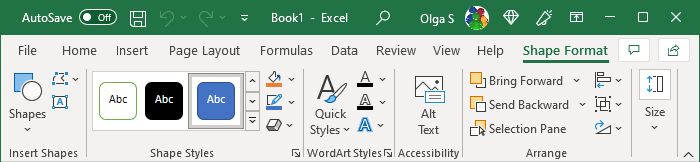When you click inside the equation, you additionally enable the Equation tab: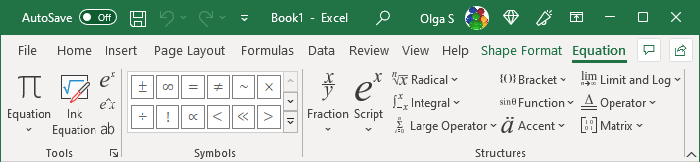• The Tools group allows to insert a new equation or control how the equation is displayed:
• The Equation dropdown list contains all predefined formulas you can insert in the current cursor position: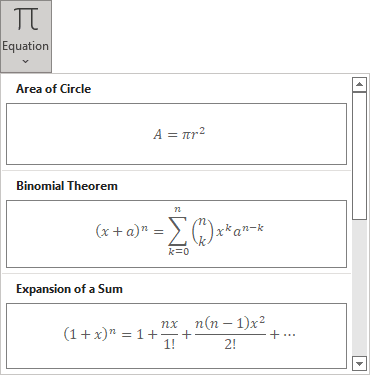• The Ink Equation button allows you to write an equation in the Math Input Control dialog box using the mouse: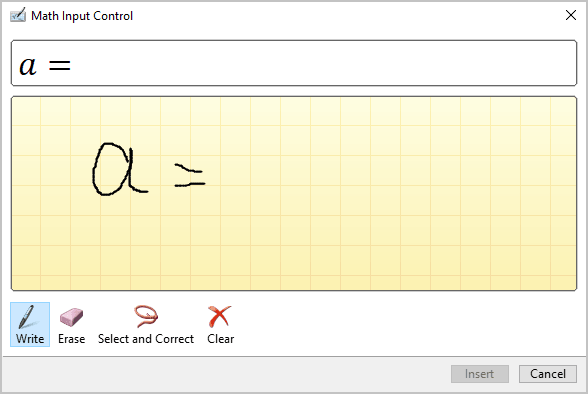See how to work with Math Input Control for more details.

• The Professional and Linear buttons allow switching between the Professional and Linear forms of the equation: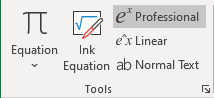and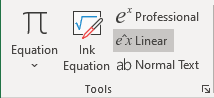The Professional form displays the equation on multiple line levels: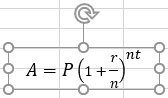where the Linear form displays it on only one line: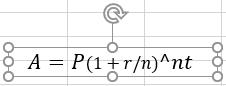• The Normal Text button allows setting font size and style: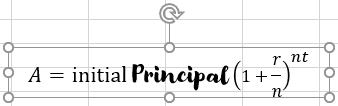Note: To be able to change the font style, the button must be highlighted for at least those characters for which you want to change the style.

• The Symbols group contains common mathematical symbols and operators that you can use in your equations.
• The Structures group contains templates for various structures that are used in equations.

As a rule, when creating an equation, a structure is added, which is then filled with other structures, text, or symbols. According to Microsoft, there is no limit to the complexity of equations.

See more about equations in Word.